# Set Times

Use this form to determine the set time required to fulfill a given water application, irrigation area, and flow rate. Learn more about the units used on this page.

Net Water Application Depth to the Soil:
Irrigation System Application Efficiency:
%
Irrigated Area:
Flow Rate:
Set Time:

## The Equation

This is the formula this calculator uses to determine the set times for an irrigation system.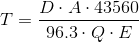Where: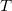= Set Time (hr)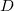= Net water application (in)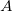= Irrigated area (acres)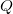= Flow Rate (gpm)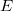= Irrigation System efficiency (%)

WSU Prosser - IAREC, 24106 N Bunn Rd, Prosser WA 99350-8694, 509-786-2226. Contact Us